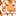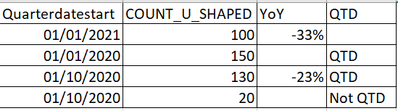# New to Qlik Sense

If you’re new to Qlik Sense, start with this Discussion Board and get up-to-speed quickly.

Announcements
cancel
Showing results for
Did you mean:Contributor III

## YoY Growth To Date

Hi,

I calculated the YoY Growth like so:

(sum({<[Qtr Diff] = {"\$(=max([Qtr Diff]))"}>}(COUNT_U_SHAPED))
-
sum({< QTD = {'QTD'},  QuarterDateStart=\$(vMaxDatePY)>}(COUNT_U_SHAPED)))

/
sum({< QTD = {'QTD'}, QuarterDateStart=\$(vMaxDatePY)>}(COUNT_U_SHAPED))

and it works great. but when changing the date, i get "-".

how can i overcome this? make it adjustable for picking different Qtr-Year?

thank you!

10 RepliesContributor III
Author

help is much appreciated 😀MVP

Hi @Shir63

Can you say what is the vMaxDatePY definition? And also, are you assigning the values to QuarterDateStart, if so, you've missed the curly brackets like below.

sum({< QTD = {'QTD'},  QuarterDateStart={'\$(vMaxDatePY)'}>}(COUNT_U_SHAPED)))

Thanks & Regards,
Mayil Vahanan RContributor III
Author

Hi Mayil,

vMaxDatePY = {"\$(=date(QuarterStart(max([QuarterDateStart])-365),'DD/MM/YYYY'))"}

and yes you are correct, this is the correct formula i copied it with a missing bracket.

thanksMVP

Hi @Shir63

You need to exclude the time filter from ur expression.

Like

sum({< QTD = {'QTD'},  QuarterDateStart={'\$(vMaxDatePY)'}, Date=, Month=, Year=>}(COUNT_U_SHAPED))

If its not works, can you share the sample file & expected o/p.

Thanks & Regards,
Mayil Vahanan RContributor III
Author

thank you for this suggestion.

when applying your suggest formula, it came back as a null.

i just need to understand why when choosing a different quarterdatestart, the result comes back as null?

here is a sample:

so for example if i change the quarterdatestart to 01/10/2020

i expect the calc to consider if it's QTD , and then sum the value of this year 100 - 130 / 130Contributor III
AuthorMVP

HI @Shir63

If you select 1/10/2020, then ur expression will change like below

vMaxDatePY gives values of 01/10/2019. i.e.

sum({< QuarterStart={"01/10/2019"}>}(COUNT_U_Shaped)), so there is no value, it display "-" / null.

Thanks & Regards,
Mayil Vahanan RContributor III
Author

no it's just an example...

in my data I do have 01/10/2019.MVP

Can you attach the sample file ?

Thanks & Regards,
Mayil Vahanan R